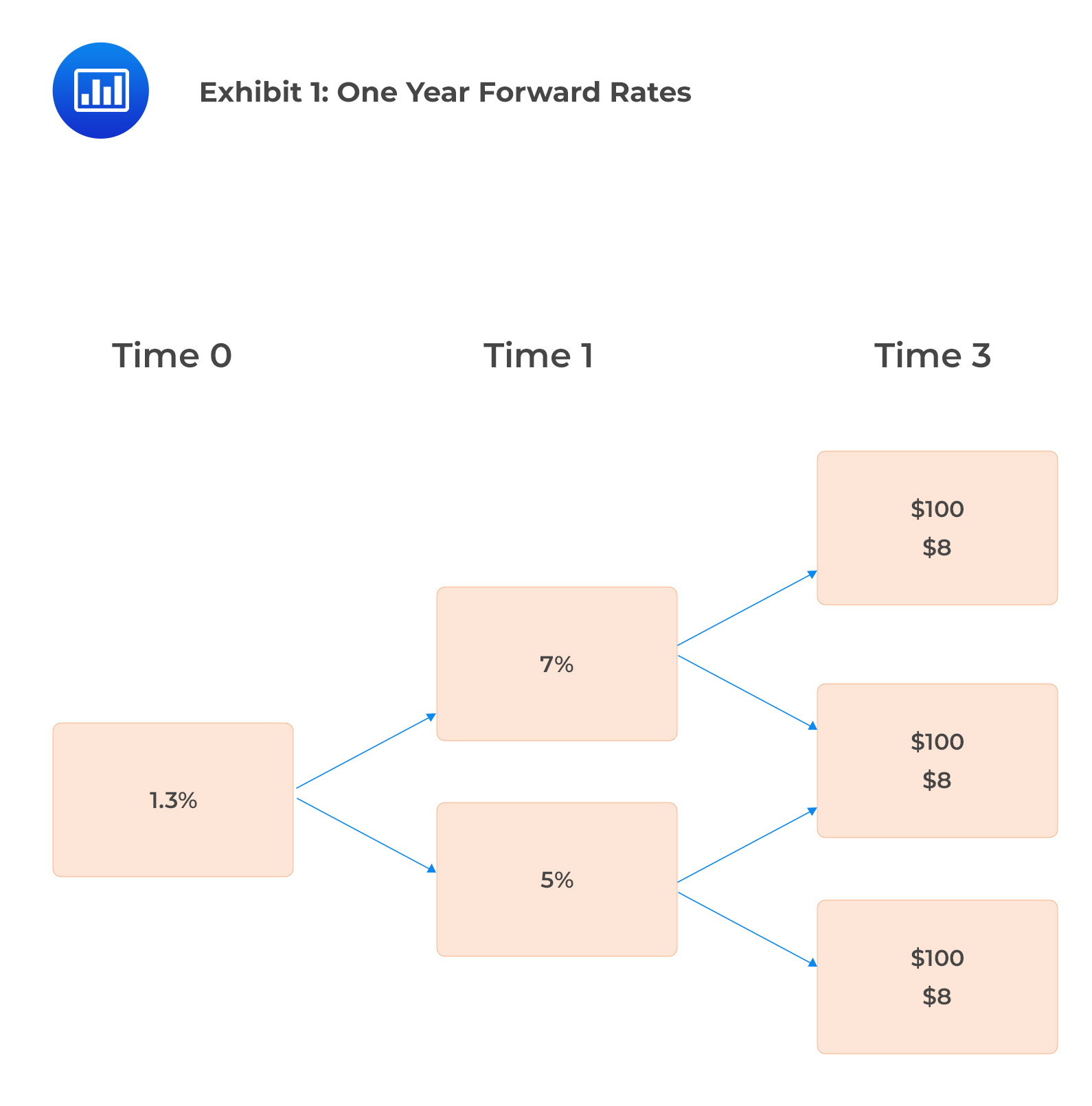# Using Arbitrage Free Framework to Value Bonds with Embedded Options

Recall that given either spot rates, forward rates, or par rates, one can determine the value of a straight bond by discounting its cashflows.

However, valuing the embedded option requires one-period forward rates. This is because we work out the value of the bond at different points in time in the future to see if the embedded option is worth exercising at those respective points in time. Thus, the binomial tree interest rates framework applies here.

#### Example: Value of Callable and Putable Bonds

We’ve been given the following yield curve:

$$\begin{array}{c|c|c} \textbf{Maturity (Years)} & \textbf{Spot Rate} & \textbf{One-Year Forward Rate} \\ \hline 1 & 1.20\% & 1.20\% \\ \hline 2 & 2.31\% & 3.44\% \\ \hline 3 & 3.35\% & 5.45\% \end{array}$$

We want to value a 5% annual coupon bond that matures in 3 years assuming that it’s:

1. Callable at par either one year or two years from today.
2. Putable at par either one year or two years from today.
3. A straight bond with zero volatility.
1. Callable Bond

$$V_{\text{2Callable}}=\frac{105}{1.0545}=99.57$$

Therefore, the bond is not called in year two as $$99.57 < 100$$.

$$V_{\text{1Callable}}=\frac{99.57+5}{1.0344}=101.09$$

The bond is called in year one as 101.09>100. The investor will only receive $100. Thus, the value of the bond in one year at this node is$100.

$$V_{\text{0Callable}}=\frac{100+5}{1.0120}=103.75$$

2. Putable Bond

$$V_{\text{2Putable}}=\frac{105}{1.0545}=99.57$$

Therefore, the put option will be exercised in year two as $$99.57 < 100$$.

Therefore, the 99.57 will be replaced by the exercise price of 100. $$V_{\text{1Putable}}=\frac{100+5}{1.0344}=101.51$$ The put option will not be exercised in year one as 101.51>100. $$V_{\text{0Putable}}= \frac{101.51+5}{1.0120}=105.25$$ 3. Straight bond \begin{align*} V_2 &=\frac{105}{1.0545}=99.57 \\ V_1 &=\frac{99.57+5}{1.0344}=101.09 \\ V_0 &=\frac{101.09+5}{1.0120}=104.83 \end{align*} 4. Value of issuer call option \begin{align*} V_{\text{Call}}& = \text{Value}_{\text{Straight}}\ – V_{\text{Callable}} \\ & =104.83-103.75=1.08 \end{align*} 5. Value of investor put option \begin{align*} V_{\text{Put}}& = V_{\text{Putable}} – V_{\text{Straight}} \\ &=105.25-104.83=0.42 \end{align*} The above calculations are shown in the following table: $$\begin{array}{l|c|c|c|c} & \textbf{Today} & \textbf{Year 1} & \textbf{Year 2} & \textbf{Year 3} \\ \hline \text{Cash flow} & & 5 & 5 & 105 \\\hline \text{One year Forward rate} & & 1.20\% & 3.44\% & 5.45\% \\ \hline \text{Value of the callable} & 103.75 & 101.10 & 99.57 & \\ \text{bond} & & & \\ \hline & & {\text{Called at }100} & \text{Not Called} & \\ \hline \text{Value of the putable} & 105.25 & 101.51 & 99.57 & \\ \text{bond} & & & \\ \hline & & \text{Not put} & {\text{Put at } 100} \\ \hline \text{Value of the straight} & 104.84 & 101.10 & 99.57 & \\ \text{bond} & & & \\ \end{array}$$ ## Question Exhibit 1 gives a binomial interest rate tree constructed assuming an interest rate volatility of 0%.Based on Exhibit 1, the value of a two-year 8% annual coupon default-free bond, callable at102 one year from today at zero volatility is closest to:

1. $104.29 2.$105.25
3. $105.67 #### Solution The correct answer is B. The bond’s value at the upper node for time 1, $$V_{1u}=0.5\left[\frac{108}{1.07}+\frac{108}{1.07}\right]=100.93$$ Thus, the bond is not called at 102. The bond’s value at the lower node for time 1, $$V_{1d}=0.5\left[\frac{108}{1.05}+\frac{108}{1.05}\right]=102.86$$ The bond is called at$102.

The value of the bond at node 0:

$$V_0=0.5\left[\frac{100.93+8}{1.04}+\frac{102+8}{1.04}\right]=105.25$$

Reading 30: Valuation and Analysis of Bonds with Embedded Options

LOS 30 (c) Describe how the arbitrage-free framework can be used to value a bond with embedded options.

Shop CFA® Exam Prep

Offered by AnalystPrepLevel I
Level II
Level III
All Three Levels
Featured Shop FRM® Exam PrepFRM Part I
FRM Part II
FRM Part I & Part II
Learn with Us

Subscribe to our newsletter and keep up with the latest and greatest tips for success
Shop Actuarial Exams PrepExam P (Probability)
Exam FM (Financial Mathematics)
Exams P & FMGMAT® Complete CourseDaniel Glyn
2021-03-24
I have finished my FRM1 thanks to AnalystPrep. And now using AnalystPrep for my FRM2 preparation. Professor Forjan is brilliant. He gives such good explanations and analogies. And more than anything makes learning fun. A big thank you to Analystprep and Professor Forjan. 5 stars all the way!michael walshe
2021-03-18
Professor James' videos are excellent for understanding the underlying theories behind financial engineering / financial analysis. The AnalystPrep videos were better than any of the others that I searched through on YouTube for providing a clear explanation of some concepts, such as Portfolio theory, CAPM, and Arbitrage Pricing theory. Watching these cleared up many of the unclarities I had in my head. Highly recommended.Nyka Smith
2021-02-18
Every concept is very well explained by Nilay Arun. kudos to you man!2021-02-13